# Kaggle Competition Tutorial: Machine Learning from the Titanic

Prepare for your first Kaggle Competition with this step-by-step tutorial.

In this tutorial, we will go through the Titanic dataset, analyze it, and submit the results to the actual competition. You have already learned how to fetch the data and import it in your Kaggle Notebook in this tutorial. Now we will be using DataCamp Workspace as the data science notebook. You will be learning how to use both tools on different platforms so that you can choose your favorite.

First, refer to the "Competitions" section in Kaggle and search for Titanic (Figure 5.4). Before going directly into the notebook, you should understand all the information shared in the "Description and Rules" sections. It is important to also check other users' notebooks, as this could give you more ideas about the solution.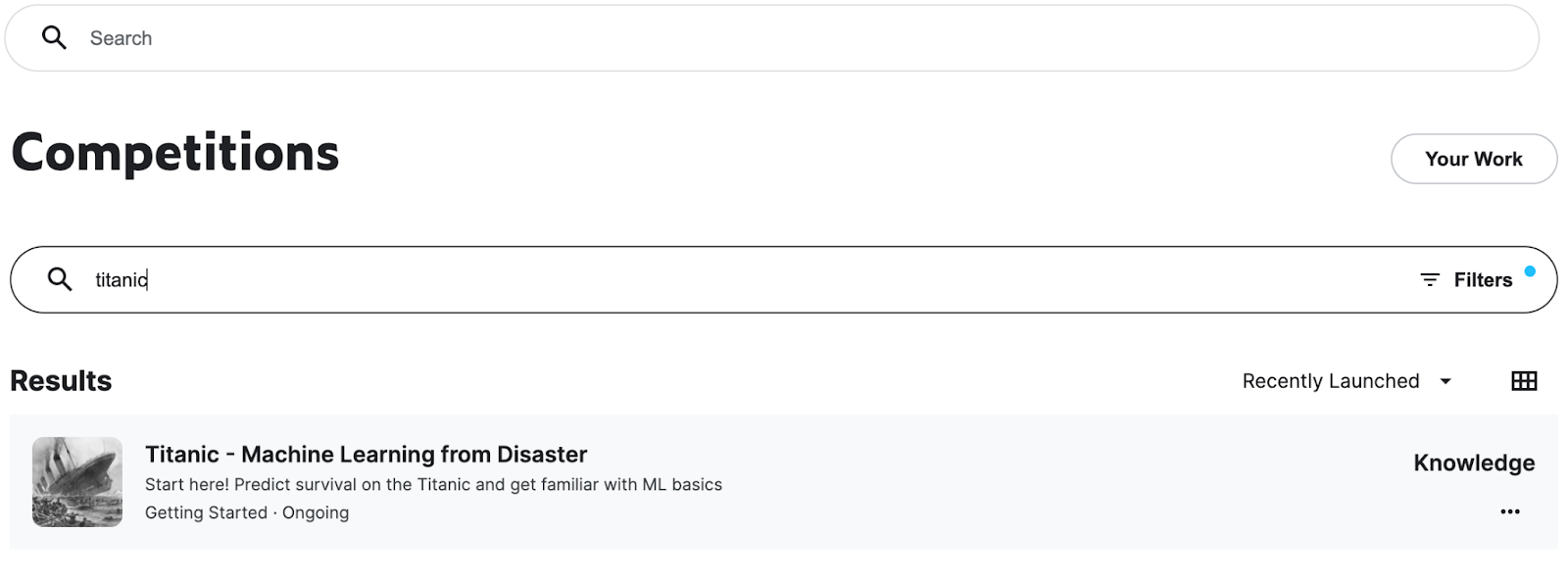Figure 5.4: Titanic - Machine Learning from Disaster

The competition is about using machine learning to create a model that predicts which passengers would have survived the Titanic shipwreck. We will be using a dataset that includes passenger information like name, gender, age, etc. There will be 2 different datasets that we will be using. The first one is titled 'train.csv'. In this dataset, besides the passenger information, there are also the ground truth values such as 'survived' and 'not survived'. In the second dataset which is titled 'test.csv', there will not be any ground truth values, since we are asked to predict those and submit our results.

The submission file will have only 2 columns: PassengerId and Survived. The submission system will produce errors if you try to upload a file with more than 2 values. Please note that you would be able to submit 10 submissions a day.

With the information provided in the "Competition Description" section, the next step would be to fetch the data to our DataCamp Workspace and analyze the data.

After downloading the files, you should be navigating to DataCamp Workspace. Please note that you need to create an account first. Creating an account and using the workspace is free.

You can easily drag and drop files on the left side of the notebook (Figure 5.6).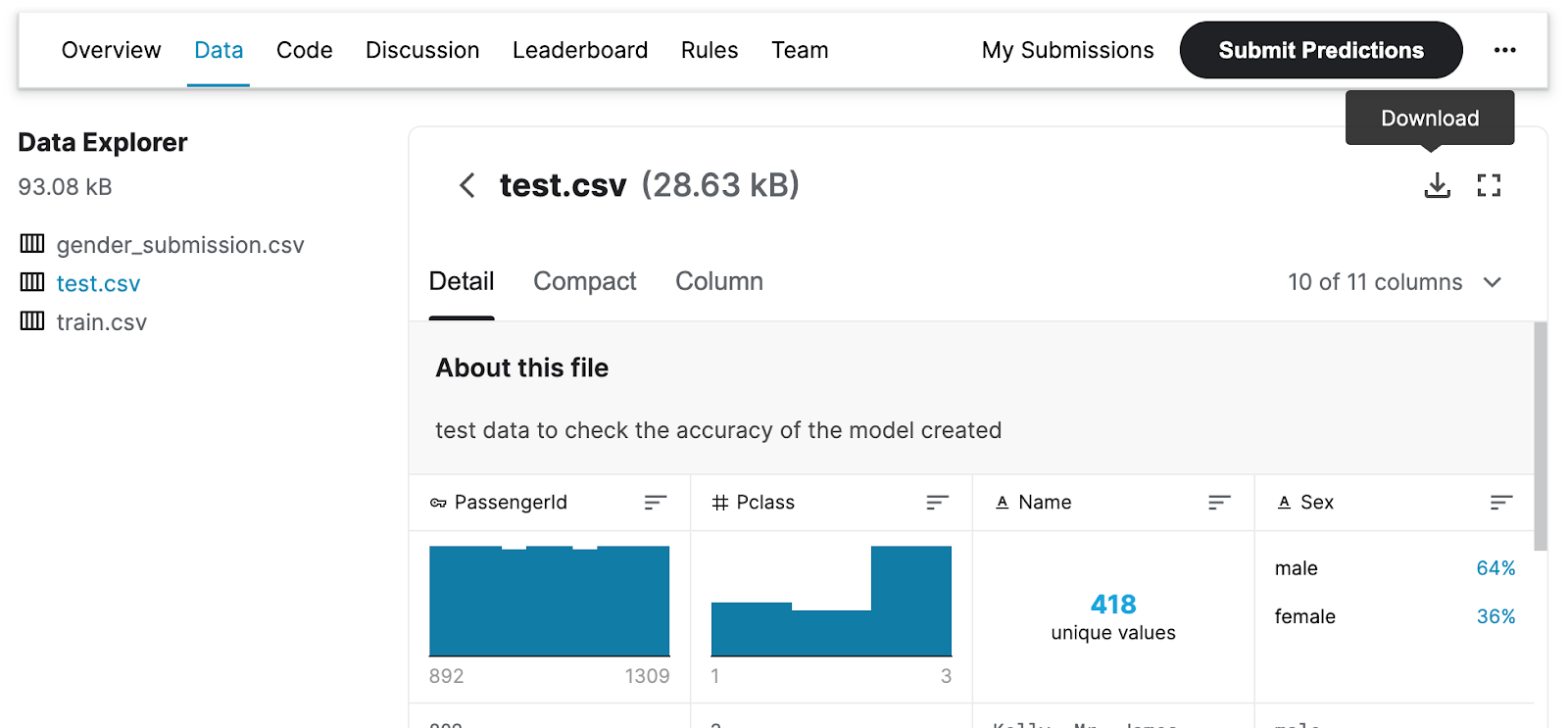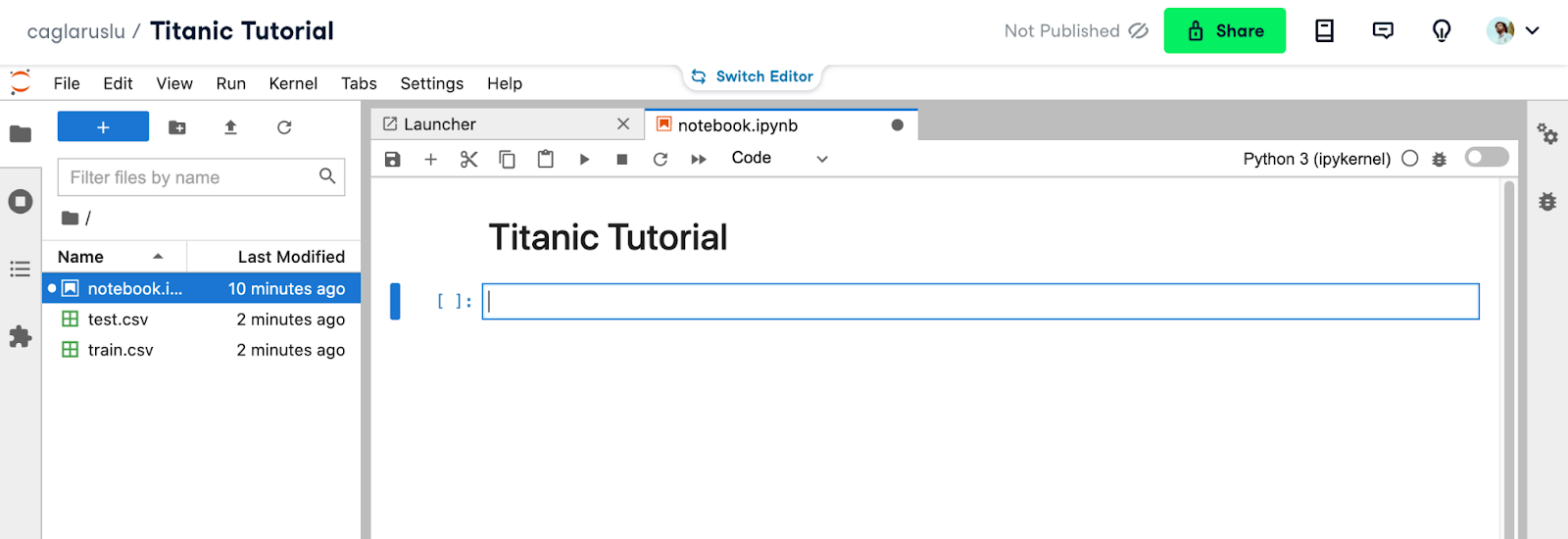Figure 5.6: Adding the Dataset to DataCamp Workspace

The next step is to check the data dictionary in the dataset description section. This will help us to decide which columns to visualize.

• survival: Survival
• pclass: Ticket class
• sex: Sex
• Age: Age in years
• sibsp: # of siblings / spouses aboard the Titanic
• parch: # of parents / children aboard the Titanic
• ticket: Ticket number
• fare: Passenger fare
• cabin: Cabin number
• embarked: Port of embarkation

## Importing the Data

You can use bash commands in jupyter notebooks by placing an exclamation mark in front of the cell. We will be checking the location of the uploaded dataset files using pwd and ls commands.

``````!pwd; ls
``````

/work/files/workspace

notebook.ipynb test.csv train.csv

Pandas is a Python library and it is used for data manipulation and data analysis processes. We have now removed Ticket, Name, and PassengerId columns since they do not have any meaningful numerical or categorical data. Now you will be able to see the rest of the features to be used.

In the next couple of lines, the training and the test dataset files are read using the read_csv method of Pandas. Note that Survived and PassengerId columns are added respectively to train and test dataframes. We will be feeding the model separately using the features in columns_to_be_added_as_features and the labels in the Survived column. But in order to make the preprocessing simpler, we can combine them for now. The test_df_matcher variable includes PassengerId as well. We will be using it to match the PassengerId with the test set predictions, to generate the CSV file which will be uploaded to Kaggle as our result.

``````import pandas as pd
``````

With the head() method, we can see the first 5 rows alongside their column names. You can define the amount of the initial rows to be shown as the parameter inside the head() method. The number of rows' values in the training set and in the test set are printed respectively in the following cell.

``````print(train_df.head())
``````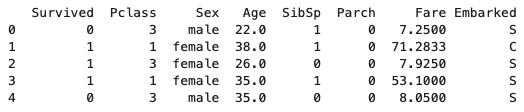Figure 5.7: Dataframe
``````print("Number of rows in training set: {}".format(len(train_df)))
print("Number of rows in test set: {}".format(len(test_df)))
``````

Number of rows in training set: 891 Number of rows in test set: 418

## Preprocessing

We should convert the type of the values to float, which will be used as features in the model. This conversion will allow us to perform some numerical operations when normalizing the data.

``````for column_title in columns_to_be_added_as_features:
if column_title in ['Embarked', "Sex"]:
continue
train_df[column_title] = pd.to_numeric(train_df[column_title], downcast="float")
test_df[column_title] = pd.to_numeric(test_df[column_title], downcast="float")

train_df["Survived"] = pd.to_numeric(train_df["Survived"], downcast="float")
``````

We need to feed the model using numerical values. In the code piece below the strings are converted into numerical values

``````train_df['Embarked'].replace('Q', 0,inplace=True)
train_df['Embarked'].replace('S', 1,inplace=True)
train_df['Embarked'].replace('C', 2,inplace=True)

test_df['Embarked'].replace('Q', 0,inplace=True)
test_df['Embarked'].replace('S', 1,inplace=True)
test_df['Embarked'].replace('C', 2,inplace=True)

train_df['Sex'].replace('male', 0,inplace=True)
train_df['Sex'].replace('female', 1,inplace=True)

test_df['Sex'].replace('male', 0,inplace=True)
test_df['Sex'].replace('female', 1,inplace=True)

``````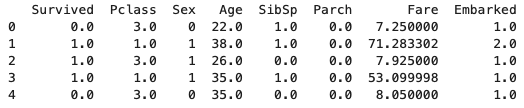Figure 5.8: Dataframe with Numerical Values

Below, all the features are normalized, which means scaled between 0 and 1 in this case.

``````#Credit: Michael Aquilina,
#https://stackoverflow.com/questions/26414913/normalize-columns-of-pandas-data-frame
def normalize(df):
result = df.copy()
for feature_name in df.columns:
max_value = df[feature_name].max()
min_value = df[feature_name].min()
result[feature_name] = (df[feature_name] - min_value) / (max_value - min_value)
return result

train_df = normalize(train_df)
test_df = normalize(test_df)

``````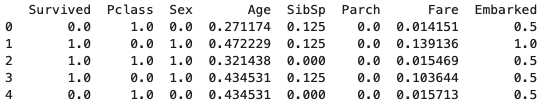Figure 5.9: Normalized Dataframe

Since the dataset will be split into training and validation, we will be shuffling the dataset first to avoid groupings in only one set.

``````#Credit: Kris, https://stackoverflow.com/questions/29576430/shuffle-dataframe-rows
train_df = train_df.sample(frac=1).reset_index(drop=True)
``````

All the empty values will be replaced with 0; this choice has been made for time-saving purposes. However, you can read this tutorial to learn how to handle missing values in machine learning.

``````train_df = train_df.fillna(0)
test_df = test_df.fillna(0)
``````

Below, the validation's ratio was set to 0.2, which means 20% of data will be used to validate it. To split the dataset into training and validation sets, we will be using Sklearn's train_test_split method. Then we will be splitting the features and labels as shown in the last 4 lines of the code piece below.

``````validation_set_ratio = 0.2
validation_set_size = int(len(train_df)*validation_set_ratio)
training_set_size = len(train_df) - validation_set_size

print("Total set size: {}".format(len(train_df)))
print("Training set size: {}".format(training_set_size))
print("Validation set size: {}".format(validation_set_size))

from sklearn.model_selection import train_test_split

train, val = train_test_split(train_df, test_size=validation_set_ratio)

train_Label = train[['Survived']]

val_Label = val[['Survived']]
``````

## Training

Support vector machines are supervised learning models that are used for classification and regression analysis. This model was chosen with the parameters below. You should be trying other models with different parameter combinations. There is no need to start and stop the code every time you want to modify a parameter or to try another model, as you can automate this process. If you are interested in Support Vector Machines, you can view the Support Vector Machines with Scikit-learn tutorial on DataCamp.

``````from sklearn.svm import SVC

SVM_KERNEL = "linear"
SVM_C = 10
SVM_GAMMA = 0.00001

svm_model = SVC(kernel = SVM_KERNEL, C = SVM_C, gamma = SVM_GAMMA)
svm_model.fit(train_X, train_Label)
``````

## Getting the Validation Set Predictions

In this part, we will be feeding the model validation set features and getting the predictions. Then we will be comparing them with the ground truth values. The Sklearn's metrics.accuracy_score method was used to make the accuracy calculation easier. The validation set accuracy is calculated as 0.799, which is a good start.

``````from sklearn import metrics

y_pred = svm_model.predict(val_X)
print("Accuracy:",metrics.accuracy_score(val_Label, y_pred))
``````

Accuracy: 0.7988826815642458

## Getting the Test Set Predictions

Now we proceed to get the predictions for the actual test set. Because there are no ground truth values for the test set, we can only know the accuracy after the predictions file is uploaded to Kaggle.

``````test_pred = svm_model.predict(test_df)
``````

## Generating the Output Dataframe

The PassengerId column is fetched from the dataframe named test_df_matcher. When it is directly returned, the type of the return value will be Series. For this reason, we will be casting it to Pandas DataFrame type. Then we will be inserting the test set predictions as the second column of the dataframe.

``````result = pd.DataFrame(test_df_matcher['PassengerId'])
``````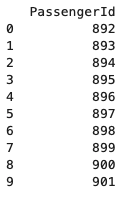Figure 5.10: PassengerId Dataframe
``````result.insert(1, "Survived", test_pred, True)
result["Survived"] = pd.to_numeric(result["Survived"], downcast="integer")
``````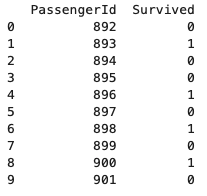Figure 5.11: Finalized Dataframe

## Generating the Output File

Finally, the output format is ready. As the last step, the index values will be removed by passing False to the index parameter. Now you can download the generated file and upload it to Kaggle. This way, you will learn the test set accuracy, after which you will be placed on the leaderboard.

``````result.to_csv("out.csv", index=False)
``````

We will now return to the competition page and click on "Submit Predictions" and then upload the predictions file. After a few seconds, you will get your test accuracy (Figure 5.12).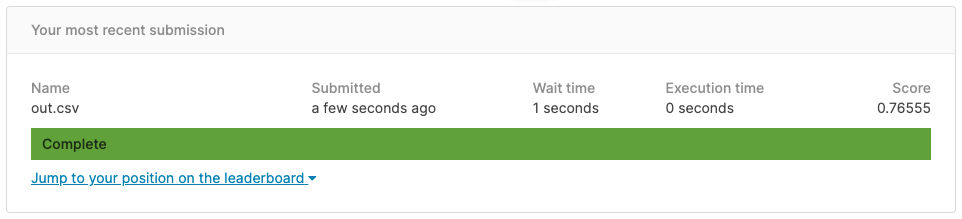Figure 5.12: Accuracy of the Test Set

## Conclusion

In this tutorial, you have learned how to analyze a dataset and submit your results to a Kaggle competition. If you enjoy doing data science competitions you can learn more about DataCamp Competitions here.